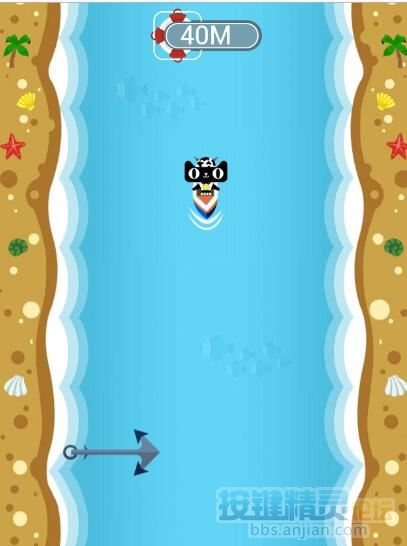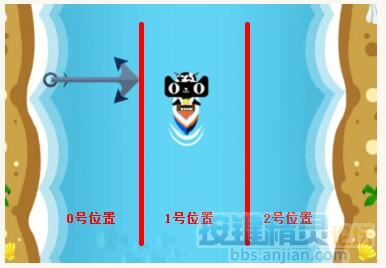1#【按键学院】安卓课程(115768679)

 在上一期的院刊中给大家带来了抢红包游戏中的【连连看】游戏的源码分析思路。大家对于这种抢红包的小游戏都比较刚兴趣，所以那今天给大家带来的是另外一款游戏的源码和分析思路——【航海喵】先定义下初始的位置为1号位置，对于铁矛的判断，左边出现了铁矛，就移动到2号位置，右边出现铁矛，就移动到1号位置。对于鲨鱼的判断，有两种朝向的鲨鱼，判断如果是朝左游的鲨鱼，则移动到2号位置，反之就移动到0号位置对于漩涡的判断，漩涡可能会出现在这三个位置，所以需要判断它的X坐标区间，从而移动小猫的位置避开

1. Function 移位(定位)
2. If 定位 = 1 Then
3. If 位置 = 0 Then
4. Swipe 169, 429, 321, 424
5. 位置 = 1
6. ElseIf 位置 = 2 Then
7. Swipe 468, 423, 321, 424
8. 位置 = 1
9. End If
10. ElseIf 定位 = 0 Then
11. If 位置 = 1 Then
12. Swipe 321, 424, 169, 429
13. 位置 = 0
14. ElseIf 位置 = 2 Then
15. Swipe 468, 423, 169, 429
16. 位置 = 0
17. End If
18. ElseIf 定位 = 2 Then
19. If 位置 = 0 Then
20. Swipe 169, 429, 468, 423
21. 位置 = 2
22. ElseIf 位置 = 1 Then
23. Swipe 321, 424, 468, 423
24. 位置 = 2
25. End If
26. End If
27. End Function

1. Dim intx,inty
2. Dim 位置 = 1 '定位初始位置
3. Function 航海喵
4. Dim 右矛 = Array(252,1100,810,1500,"A38369","2|21|836A56,92|8|A38369,16|-17|5C2E16")
5. Dim 左矛 = Array(252,1100,810,1500,"A38369","-3|14|836A56,-18|-20|5C2E16,-144|6|A7866C")
6. Dim 左鲨鱼 = Array(252,1100,810,1500,"938D88","34|4|1D27A5,-5|10|1D27A5,-18|5|FFFFFF")
7. Dim 右鲨鱼 = Array(252,1100,810,1500,"938D88","-32|1|1D27A5,12|7|1D27A5,24|4|FFFFFF")
8. Dim 漩涡 = Array(252,1100,810,1500,"C5A126","-8|-42|C5A126,-37|-8|C5A126,3|12|C5A126")
9. Do
10. KeepCapture
11. If FindColorEx(右矛) Then
12. Call 移位(0)
13. ElseIf FindColorEx(左矛) Then
14. Call 移位(2)
15. ElseIf FindColorEx(左鲨鱼) Then
16. Call 移位(2) '鲨鱼朝左游，小猫移动到右边
17. ElseIf FindColorEx(右鲨鱼) Then
18. Call 移位(0) '鲨鱼朝右游，小猫移动到左边
19. ElseIf FindColorEx(漩涡) Then
20. If intx < 257 Then
21. Call 移位(2) '漩涡在左边，小猫移动到右边
22. ElseIf intx > 392
23. Call 移位(0) '漩涡在右边，小猫移动到左边
24. Else
25. Call 移位(0) '漩涡在中间，小猫移动到右边
26. End If
27. End If
28. Delay 100
29. Loop
30. End Function

31. Function 移位(定位)
32. If 定位 = 1 Then
33. If 位置 = 0 Then
34. Swipe 169, 429, 321, 424
35. 位置 = 1
36. ElseIf 位置 = 2 Then
37. Swipe 468, 423, 321, 424
38. 位置 = 1
39. End If
40. ElseIf 定位 = 0 Then
41. If 位置 = 1 Then
42. Swipe 321, 424, 169, 429
43. 位置 = 0
44. ElseIf 位置 = 2 Then
45. Swipe 468, 423, 169, 429
46. 位置 = 0
47. End If
48. ElseIf 定位 = 2 Then
49. If 位置 = 0 Then
50. Swipe 169, 429, 468, 423
51. 位置 = 2
52. ElseIf 位置 = 1 Then
53. Swipe 321, 424, 468, 423
54. 位置 = 2
55. End If
56. End If
57. End Function

58. Function FindColorEx(ColorArr)
59. FindMultiColor ColorArr(0), ColorArr(1), ColorArr(2), ColorArr(3), ColorArr(4), ColorArr(5), 0, 0.9, intX, intY
60. If intX > -1 Then
61. FindColorEx = True
62. Else
63. FindColorEx = False
64. End If
65. End Functionhttp://bbs.anjian.com/showtopic-584228-1.aspx